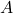# Structural Calculations for Beams Using Calculus via Sympy Jul 2022

Something about calculus and Sympy is lovable
I find it effective to use closed form solutions and to be able to develop/verify special cases using well-known (and well worn) formulas.

I built a Jupyter notebook. Here is my summary of the contents.

1. Given a beam with fixed ends and a single concentrated load:
(a) Write the equation for the reactions,,,, and,
(b) Plot the reactionfor all possible locations of,
(c) Build a function, using indefinite integration to determineandfor a partial uniform moment,,
(d) Build a function, using definite integration to determineandfor a partial uniform moment,,
(e) Consider the point load,, located at a distance,, from the left support. What locationwould result in the maximum moment at the left support?,
(f) Consider a partial uniform load, applied to the beam only over a distance L/2, what location of the uniform load would result in the maximum moment at the left support?,
(g) Determine the solution offor any arbitrary partial uniform loading,,
(h) Determine the solution offor any arbitrary partial uniform loading,,
(Bonus 1) Determine the solution offor various ‘checkered’ uniform loadings,,
(Bonus 2) Determine the solution offor various ‘checkered’ uniform loadings,.

My Jupyter Notebook

Click to see notebook PDF.

Live Charts

Click to seeplot; hover over data points to see data values; use finger or mouse to select rectangular area of interest

Click to seeplot; hover over data points to see data values; use finger or mouse to select rectangular area of interest